# Physics Symbols and Meanings

Physics is the branch of science that deals with studying the properties and nature of matter and energy. Six branches in physics cover the study of small objects using quantum mechanics to study the entire universe. From here, learn about the physics symbols that represent quantities in all branches of physics.

## 1. What Are Physics Symbols

The subject of physics covers a wide range of physical quantities as it deals with studying every aspect related to the physical world. There are lots of calculations involved with the study of energy and matter. We use physics symbols or notations to represent various quantities in physics. It makes it easy to perform calculations and understand the basic concepts.

Physicals deal with various quantities; therefore, there are multiple types of symbols and notations. The most familiar characters used in physics are Greek alphabets that come with one or two letters from the name of quantities. The other one is math symbols that we use to perform calculations. We use SI units to represent coefficients and quantities in physics.

We use a variety of symbols to denote parameters, constants and equations in physics. It helps us better understand complex calculations and makes it easier to make representations. Although using symbols makes a lot of things clear, it can also complicate things if you don’t know the exact signs and their uses. It is because some symbols represent more than one quantity in physics.

## 2. Physics Symbols Explained

Symbols are a cornerstone in representing physics. There are a large variety of quantities in the six branches of physics. That is why listing all types of physics symbols together only makes everything more complex. Grouping symbols concerning their uses in various branches of physics makes it easy to identify and understand them. Now we are going to take a look at the most used symbols and their uses.

### 2.1 Physics Symbols and Icons in Mechanics

Mechanics is the branch of physics that deals with the study of the motion of objects. It studies the relationship between the force and motion of various objects. It identifies the reasons and properties of motion related to object matter in a specific time and applied force. It tells us whether an object is moving due to some force or just displacing it in a particular environment.

Mechanics coves various physical phenomena such as gravity, kinematics and laws of motions. We use multiple types of objects to experiment and find out more about their mechanics. When it comes to studying gravity and its effects, we use things with different weights. We use springs and pulleys to experiment with the properties of various types of motion. Following are some of the symbols and icons we use in mechanics.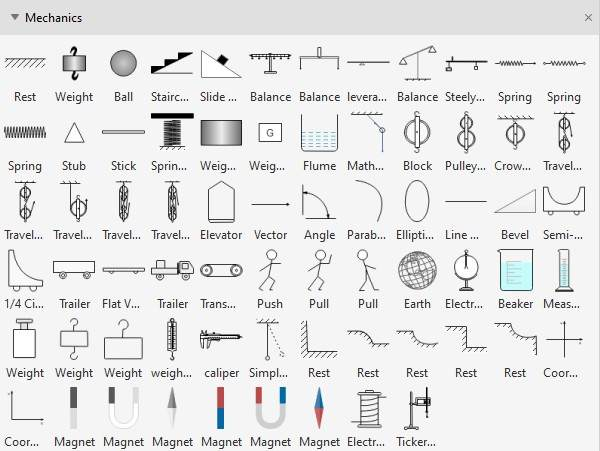Source: EdrawMax Online

Mechanics symbols represent quantities like speed, mass, momentum, pressure or power. When calculating the motion of an object in any given environment, we use mechanic symbols to represent constants and SI units. Scalers and vectors are the two types of physical quantities in mechanics. We use different units to represent these quantities. Following are some units we use in mechanics.

 Physical Quantity Symbol(s) Scalar / Vector SI Unit Velocity v Vector Meter per second m/s Acceleration a Vector meters per second squared (m/s2) Momentum m Vector Kilogram meter per second Kg m/s Time T Scaler Second s Torque T Vector Newton meter Nm Power P Scaler Watt W Force F Vector Newton N Angular Momentum α Vector Radian per second squared rad/s2 Energy E Scaler Joule J Pressure P Scaler Pascal P Work W Scaler Joule J Inertia I Scaler Kilogram meter squared Kg m2 Kinetic Energy K Scaler Joule J Potential Energy P Scaler Joule J Friction F Vector Newton N Coefficient of Friction µ Scaler No unit

### 2.2 Physics Symbols and Icons in Waves & Optics

Physical optics is the branch of chemistry that deals with various phenomena regarding rays of lights and their approximation. Waves and optics both cover the studies related to reflection or refraction. It tells us about the interference and polarization of the rays of light through physical objects. On the other hand, wave optics deals with the behaviour of light when it comes in contact with any small or large object.

When it comes to studying light and its behaviour, we use special equipment. We use various types of glass lenses, convex, concave or others, to analyse the properties of light rays. We use prisms, bulbs, candles and beams of light to do experiments. We also use the structure of the human eye for in-depth research. Here are some commonly used waves and optics icons and symbols.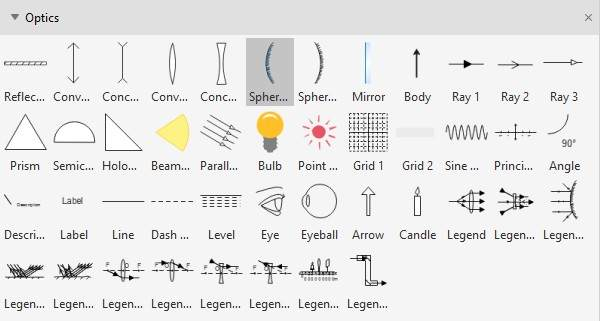Source: EdrawMax Online

In physical optics, we calculate the wavelength, frequency other properties of light waves. Sometimes we have to study angular moments of light, or sometimes we study rays moving in a straight path. That is why we use specific symbols and notations to keep everything simple. These are some of the most used characters and their SI units.

 Physical Quantity Symbol(s) Scalar / Vector SI Unit Wavelength λ Scalar Meter m Focal length f Scaler Meter m Magnification M Scaler No unit Absolute refraction index n Scaler No unit Intensity I Scaler Watt per meter squared W/m2 Level L Scaler Decibel dB Wave speed c, u Scaler Meter per second m/s

### 2.3 Physics Symbols in Thermodynamics

Thermodynamics is the branch of physics that deals with the study of energy conversion in a system. In simple words, it tells us how some type of energy, for example, heat moves from one body to another and converts into another form of energy such as kinetic energy. In thermodynamics, we use symbols to represent heat, work, energy and their relationship. Here are some of the commonly used thermodynamic symbols in physics.

 Physical Quantity Symbol(s) Scalar / Vector SI Unit Temperature T Scalar Kelvin K Heat Q Scaler Joule J Specific Heat c Scaler Joule per kilogram Kelvin J/kg K Latent Heat L Scaler Joule per kilogram J/kg Linear Expansion α Scaler Kelvin Inverse K−1 Volume Expansion β Scaler Kelvin Inverse K−1 Thermal Conductivity k Scaler Watt per meter Kelvin W/m K Entropy S Scaler Joule per Kelvin J/K Heat Flow Rate P Scaler Watt W Internal Energy U Scaler Joule J

### 2.4 Physics Symbols in Electricity & Magnetism

Electromagnetism is the branch of physics that deals with electromagnetic force. It is the study of forces and interaction between two or more electromagnetically charged particles. This branch of physics involves the study of magnetic fields and the behaviour of particles in an electric field. We study various phenomena like electromagnetic induction, power, resistance and capacitance of particles. Here are some commonly used icons for the representation of electromagnetism in physics.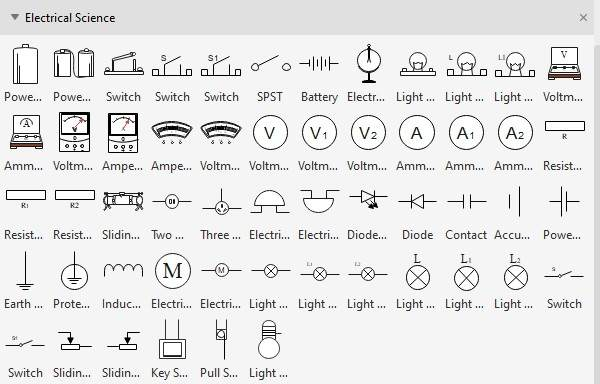Source: EdrawMax Online

The study of magnetic and electric fields involves various variables, constants and equations. It usually requires complex calculations but using SI units and physics symbols make it easier to understand. We use characters to represent electricity and magnetism between particles and their properties. These are some of the most used quantities, their signs and SI units.

 Physical Quantity Symbol(s) Scalar / Vector SI Unit Charge q,Q Scalar Coulomb C Magnetic Field B Scaler Tesla Electric Field E Vector Newton per coulomb N/C Capacitance C Scaler Farad F Inductance L Scaler Henry H Current I Scaler Ampere A Resistance R Scaler Resistance Ω Electric Potential Difference V Scaler Volt V

### 2.5 Physics Symbols in Time & Space

Physics is the only branch of science that deals with the study of time as a unique quantity. Time is an essential part of lots of equations and calculations in physics. It refers to the interval during which a change occurs in the system. Space is the unit that represents the three-dimensional plane in which various objects exist and events occur. Following are the units we use to depict Time and Space in Physics.

 Physical Quantity Symbol(s) Scalar / Vector SI Unit Frequency f Scalar Hz Angular Frequency w Scaler Rad/s Area A Scaler Meter squared Volume V Scaler Meter Cube Time t Scaler Second Periodic Time T Scaler Second Length L Scaler Meter Diameter D Scaler Meter Circumference C Scaler Meter Cartesian coordinates x, y, z Scaler No unit Cartesian unit vectors î, ĵ, k̂ Vector No unit Spherical unit vector r̂, θ̂, φ̂ Vector No unit Spherical coordinates r, θ, φ Scaler Meter, Radian Cylindrical coordinates r, θ, z Scaler Meter, Radian Cylindrical unit vectors r̂, θ̂, ẑ Vector No unit

## 3. Tips for Using Physics Symbols

If you want to make a physics presentation, you need Physics symbols to make it clear and easy to understand. We recommend EdrawMax Online to make your presentation. There are many physics icons and logos in its library that you can use for your projects. Follow these steps to get straight to physics symbols in EdrawMax.

1. If you are writing a math equation for physics, go to EdrawMax Online> Insert>Math Equation.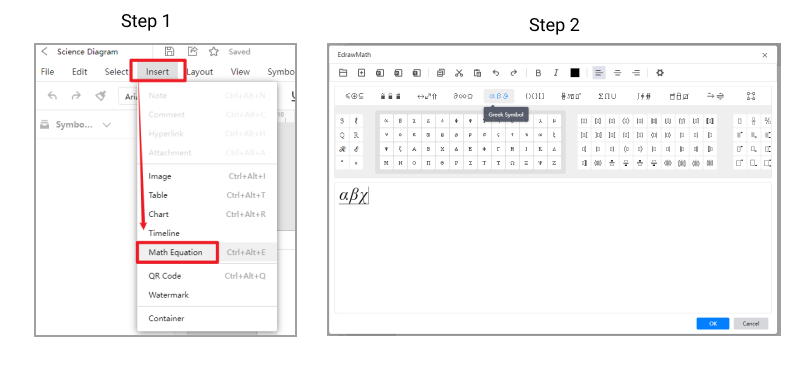2. For projects and presentations, go to the library and get the physics icons you need to make a diagram for your project.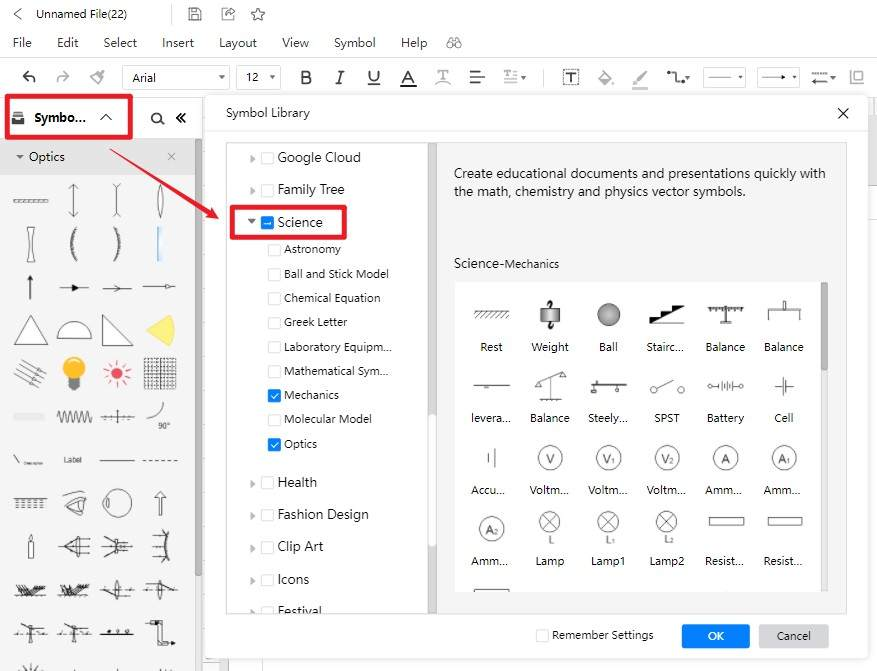3. If you don't find your required symbols or icons, you can draw them there or insert them outside. Watch this video to learn more about it YouTube.

## 4. Conclusion

There are six branches in physics, and each comes with various symbols and units to represent quantities. Suppose you are doing a project or doing some calculations related to physics, then you can do it correctly after learning about physical symbols and their uses. The same symbols represent different quantities in physics, so make sure to use icons to make your presentation and science diagram clear. EdrawMax Online is an excellent tool because of its wide support and extensive features like comprehensive symbols library, science graphic organizer examples, export in multiple formats, and import of symbols and libraries. It is available for free online and desktop with maximum feature support.

## Create and share 280+ diagrams with EdrawMax Online

Related Articles